# Cylinder + surface area - math problems

#### Number of problems found: 58

• The surfaceThe surface of the cylinder is 1570 cm2, its height is 15 cm. Find its volume and radius of the base.
• Cans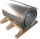How many m2 of metal sheet is needed to produce 20,000 cans in the shape of a cylinder with a base radius and a height of 5 cm.
• What is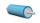What is the height of a cylinder whose surface size is 602.88 cm2 and the content of its shell is 376.8 cm2?
• The cylinder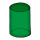In a rotating cylinder it is given: the surface of the shell (without bases) S = 96 cm2 and the volume V = 192 cm cubic. Calculate the radius and height of this cylinder.
• Rotary cylinderIn the rotary cylinder it is given: surface S = 96 cm2 and volume V = 192 cm cubic. Calculate its radius and height.
• Surface and volumeCalculate the surface and volume of a cylinder whose height is 8 dm and the radius of the base circle is 2 dm
• Water reservoir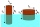What is the weight of a metal reservoir - cylinder with a diameter of 2 m and a length 8 m, if 1 m2 of sheet metal weighs 100 kg?
• Fountain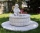The stone fountain, which has the shape of a cylinder with a diameter of 3 m, is 70 cm deep. How many m2 of stone is wetted with water?
• Shell area cyThe cylinder has a shell content of 300 cm square, while the height of the cylinder is 12 cm. Calculate the volume of this cylinder.
• The cylinderThe cylinder has a surface area of 300 square meters, while the cylinder's height is 12 m. Calculate the volume of this cylinder.
• Metal sheets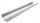How much metal sheet is needed to produce 8 gutters 4 m long and 12 cm in diameter? During production, joints calculate at 3% of total consumption.
• The hollow cylinderThe hollow cylinder has a height of 70 cm, an outer diameter of 180 cm, and an inner diameter of 120 cm. What is the surface of the body, including the area inside the cavity?
• Winch drum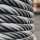Originally an empty winch drum with a diameter of 20 cm and a width of 30 cm on the rescue car, he started winding a rope with a thickness of 1 cm beautifully from edge to edge. The winch stopped after 80 turns. It remains to spin 3.54m of rope (without h
• Diameter = heightThe cylinder's surface, the height of which is equal to the diameter of the base, is 4239 cm square. Calculate the cylinder volume.
• Two bodiesThe rectangle with dimensions 8 cm and 4 cm is rotated 360º first around the longer side to form the first body. Then, we similarly rotate the rectangle around the shorter side b to form a second body. Determine the ratio of surfaces of the first and seco
• Cylinder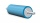The 1.8m cylinder contains 2000 liters of water. What area (in dm2) of this container is the water?
• Surface of the cylinderCalculate the surface of the cylinder for which the shell area is Spl = 20 cm2 and the height v = 3.5 cm
• Gutter pipe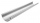How many m² of sheet metal is required to produce a 12 m long and 18 cm wide gutter, if 7% bend is required?
• The cylinder 2Find the volume and the lateral area of a cylinder of height 12 inches and a base radius of 4 inches.
• Spherical capPlace a part of the sphere on a 4.6 cm cylinder so that the surface of this section is 20 cm2. Determine the radius r of the sphere from which the spherical cap was cut.

Do you have an exciting math question or word problem that you can't solve? Ask a question or post a math problem, and we can try to solve it.

We will send a solution to your e-mail address. Solved examples are also published here. Please enter the e-mail correctly and check whether you don't have a full mailbox.

Cylinder Problems. Examples for the calculation of the surface area of ​​the solid object .